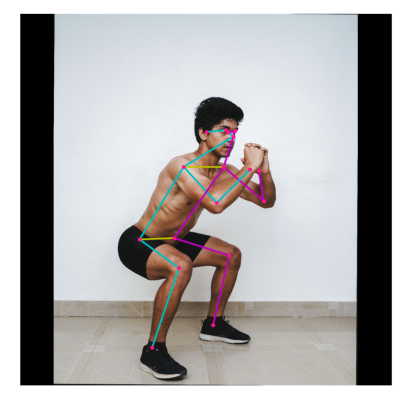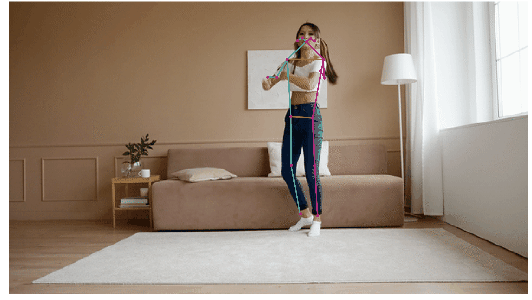Help protect the Great Barrier Reef with TensorFlow on Kaggle

# MoveNet: Ultra fast and accurate pose detection model.

MoveNet is an ultra fast and accurate model that detects 17 keypoints of a body. The model is offered on TF Hub with two variants, known as Lightning and Thunder. Lightning is intended for latency-critical applications, while Thunder is intended for applications that require high accuracy. Both models run faster than real time (30+ FPS) on most modern desktops, laptops, and phones, which proves crucial for live fitness, health, and wellness applications.This Colab walks you through the details of how to load MoveNet, and run inference on the input image and video below.

# Human Pose Estimation with MoveNet

## Visualization libraries & Imports

````pip install -q imageio`
`pip install -q opencv-python`
`pip install -q git+https://github.com/tensorflow/docs`
```
``````import tensorflow as tf
import tensorflow_hub as hub
from tensorflow_docs.vis import embed
import numpy as np
import cv2

# Import matplotlib libraries
from matplotlib import pyplot as plt
from matplotlib.collections import LineCollection
import matplotlib.patches as patches

# Some modules to display an animation using imageio.
import imageio
from IPython.display import HTML, display
``````

## Load Model from TF hub

``````model_name = "movenet_lightning"

if "tflite" in model_name:
if "movenet_lightning_f16" in model_name:
input_size = 192
elif "movenet_thunder_f16" in model_name:
input_size = 256
elif "movenet_lightning_int8" in model_name:
input_size = 192
elif "movenet_thunder_int8" in model_name:
input_size = 256
else:
raise ValueError("Unsupported model name: %s" % model_name)

# Initialize the TFLite interpreter
interpreter = tf.lite.Interpreter(model_path="model.tflite")
interpreter.allocate_tensors()

def movenet(input_image):
"""Runs detection on an input image.

Args:
input_image: A [1, height, width, 3] tensor represents the input image
pixels. Note that the height/width should already be resized and match the
expected input resolution of the model before passing into this function.

Returns:
A [1, 1, 17, 3] float numpy array representing the predicted keypoint
coordinates and scores.
"""
# TF Lite format expects tensor type of uint8.
input_image = tf.cast(input_image, dtype=tf.uint8)
input_details = interpreter.get_input_details()
output_details = interpreter.get_output_details()
interpreter.set_tensor(input_details['index'], input_image.numpy())
# Invoke inference.
interpreter.invoke()
# Get the model prediction.
keypoints_with_scores = interpreter.get_tensor(output_details['index'])
return keypoints_with_scores

else:
if "movenet_lightning" in model_name:
input_size = 192
elif "movenet_thunder" in model_name:
input_size = 256
else:
raise ValueError("Unsupported model name: %s" % model_name)

def movenet(input_image):
"""Runs detection on an input image.

Args:
input_image: A [1, height, width, 3] tensor represents the input image
pixels. Note that the height/width should already be resized and match the
expected input resolution of the model before passing into this function.

Returns:
A [1, 1, 17, 3] float numpy array representing the predicted keypoint
coordinates and scores.
"""
model = module.signatures['serving_default']

# SavedModel format expects tensor type of int32.
input_image = tf.cast(input_image, dtype=tf.int32)
# Run model inference.
outputs = model(input_image)
# Output is a [1, 1, 17, 3] tensor.
keypoints_with_scores = outputs['output_0'].numpy()
return keypoints_with_scores
``````

## Single Image Example

This session demonstrates the minumum working example of running the model on a single image to predict the 17 human keypoints.

````curl -o input_image.jpeg https://images.pexels.com/photos/4384679/pexels-photo-4384679.jpeg --silent`
```
``````# Load the input image.
image_path = 'input_image.jpeg'
image = tf.image.decode_jpeg(image)
``````

### Run Inference

``````# Resize and pad the image to keep the aspect ratio and fit the expected size.
input_image = tf.expand_dims(image, axis=0)

# Run model inference.
keypoints_with_scores = movenet(input_image)

# Visualize the predictions with image.
display_image = tf.expand_dims(image, axis=0)
display_image, 1280, 1280), dtype=tf.int32)
output_overlay = draw_prediction_on_image(
np.squeeze(display_image.numpy(), axis=0), keypoints_with_scores)

plt.figure(figsize=(5, 5))
plt.imshow(output_overlay)
_ = plt.axis('off')
``````## Video (Image Sequence) Example

This section demonstrates how to apply intelligent cropping based on detections from the previous frame when the input is a sequence of frames. This allows the model to devote its attention and resources to the main subject, resulting in much better prediction quality without sacrificing the speed.

### Cropping Algorithm

````wget -q -O dance.gif https://github.com/tensorflow/tfjs-models/raw/master/pose-detection/assets/dance_input.gif`
```
``````# Load the input image.
image_path = 'dance.gif'
image = tf.image.decode_gif(image)
``````

### Run Inference with Cropping Algorithm

``````# Load the input image.
num_frames, image_height, image_width, _ = image.shape
crop_region = init_crop_region(image_height, image_width)

output_images = []
bar = display(progress(0, num_frames-1), display_id=True)
for frame_idx in range(num_frames):
keypoints_with_scores = run_inference(
movenet, image[frame_idx, :, :, :], crop_region,
crop_size=[input_size, input_size])
output_images.append(draw_prediction_on_image(
image[frame_idx, :, :, :].numpy().astype(np.int32),
keypoints_with_scores, crop_region=None,
close_figure=True, output_image_height=300))
crop_region = determine_crop_region(
keypoints_with_scores, image_height, image_width)
bar.update(progress(frame_idx, num_frames-1))

# Prepare gif visualization.
output = np.stack(output_images, axis=0)
to_gif(output, fps=10)
``````[]
[]